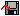# Settings - Calculations

The Calculations tab is only available in the operating modes velocity, with ramps and path control.

This dialog calculates the motor parameters from the application parameters (e.g. register values of the stepper motor terminal) and then displays them. This calculations are also available as an Excel table. To use this Excel table, macro execution has to be enabled in Excel!Excel table (download)

## Application parameters:

• Maximum coil current (RP0.R35)
Current limit for winding A and B. The preset value is calculated from the maximum coil current A (in %) entered in the Settings dialog and the maximum rated current of the stepper motor terminal.
• Feed per revolution
Enter a value (parameter relating to the connected mechanical system) that is then used for calculating the motor parameters. This value is not transferred to the stepper motor terminal and is only used for illustrating the motor parameters.
• Full steps per revolution (motor) (RP0.R33)
The value from the Settings dialog is preset.
• Increments per revolution (encoder), KL2541 only (RP0.R34)
The value from the Settings dialog is preset.
• Min. velocity (R38)
The value from the Settings dialog is preset. This value is used for path control mode and transferred to the Commissioning dialog.
• Max. velocity (R39)
The value from the Settings dialog is preset. This value is used for path control mode and transferred to the Commissioning dialog.
• Max. acceleration (R40)
The value from the Settings dialog is preset. This value is used for path control mode and transferred to the Commissioning dialog.
• Max. deceleration (R58)
The value from the Settings dialog is preset. This value is used for path control mode and transferred to the Commissioning dialog.
• Process data
This field can be used for entering a process data (decimal) that is then used for calculating the resulting velocity based on the specified motor parameters. This process data is not transferred to the stepper motor terminal and is only used for illustrating the motor parameters.

## Motor parameter:

• Coil current
Specifies the maximum coil current in percent (RP0.R35 and RP0.R36) of the maximum rated current of the stepper motor terminal.
• Min. velocity Coasting velocity (in operating mode path control)
• as feed rate
• in full steps per second
• as decimal register value (R38)
• Max. velocity
Representation of the maximum velocity
• as feed rate
• in full steps per second
• as decimal register value (R39)
• Max. acceleration
Representation of the maximum acceleration
• as feed rate
• in full steps per second squared
• as decimal register value (R40)
• Max. deceleration
Representation of the maximum acceleration
• as feed rate
• in full steps per second squared
• as decimal register value (R58)
• Recommended step size
This is the recommended value for the smallest possible step size with given application parameters. The user can increase the step size if necessary. However, please note that specifying the velocity via the process data requires a new calculation.
• Full step angle
Specifies the resulting angle for a full step.
• Microstep angle
Specifies the resulting angle for a microstep.
• Microsteps per revolution
Specifies the number of microsteps required for a complete turn of the motor shaft.
• Current velocity
Indicates the resulting velocity for value entered under process data and the given application parameters.
• Scaling factor (SF)
Calculated based on the formula:
SF = feed rate/revolution / (full steps/revolution x step size)
• Reference velocity (vref)
Calculated based on the formula:
vref = SF x DataOUTmax x 3.812951 = SF x 32767 x  3.812951
(See chapter Calculation of the velocity)